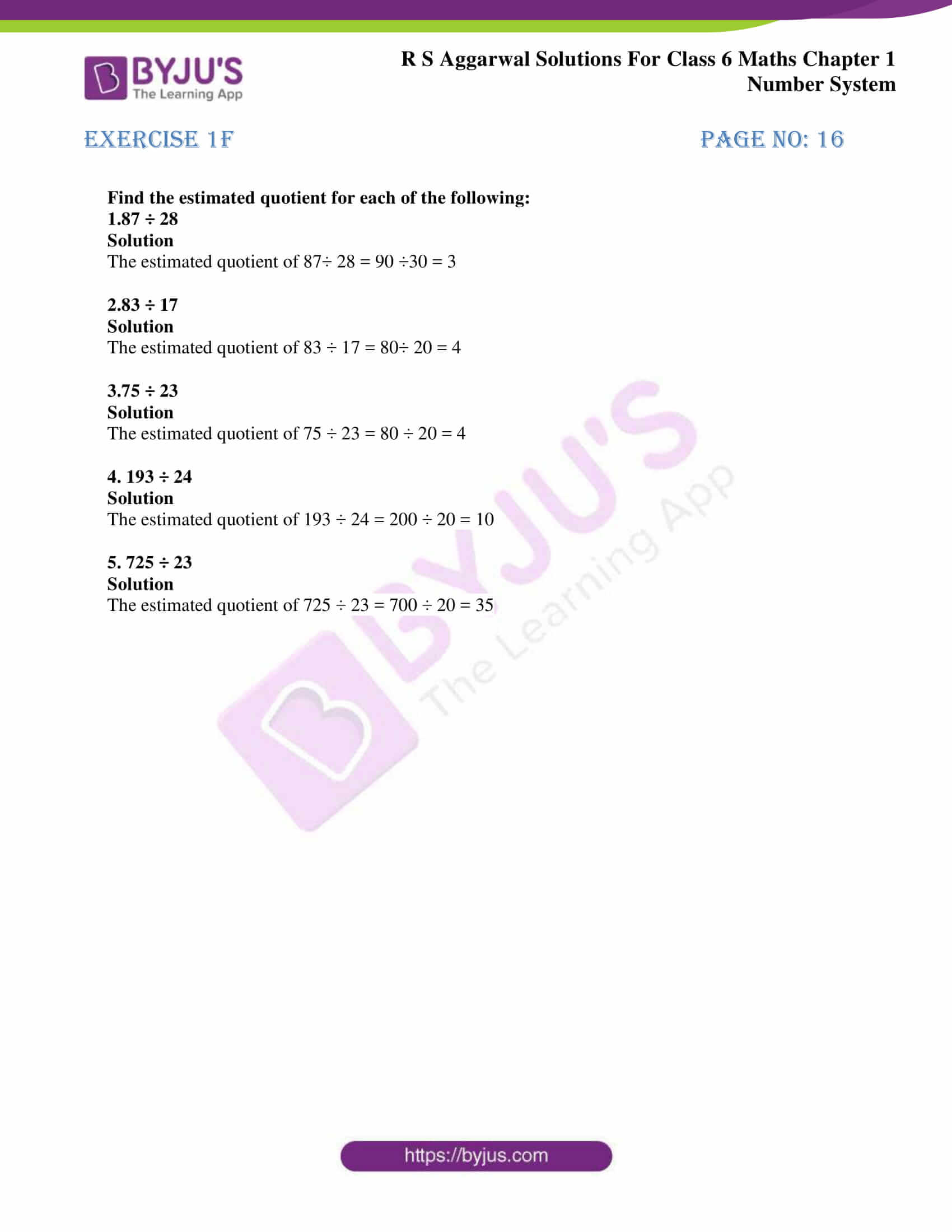# RS Aggarwal Solutions for Class 6 Chapter 1 Number System Exercise 1F

RS Aggarwal Solutions for Class 6, Chapter 1, Number System Exercise 1F are available here in PDF format. By regularly practising RS Aggarwal Solutions, students gain conceptual knowledge and become experts in Mathematics. This also helps them to score high marks in examinations as per the CBSE board. Exercise 1F of RS Aggarwal solutions has the problems based on the topic Estimating the quotients and steps followed in solving them.

At BYJU’S, we have faculty members with experience in the respective field to solve exercise wise problems of RS Aggarwal textbook. Students who dream to ace the examination easily can refer RS Aggarwal Solutions for Class 6 Chapter 1 Number system Exercise 1F.

## Download PDF of RS Aggarwal Solutions for Class 6 Chapter 1 Number System Exercise 1F### Access answers to Maths RS Aggarwal Solutions for Class 6 Chapter 1 Number System Exercise 1F

Find the estimated quotient for each of the following:

1.87 ÷ 28

Solution

The estimated quotient of 87÷ 28 = 90 ÷30 = 3

2.83 ÷ 17

Solution

The estimated quotient of 83 ÷ 17 = 80÷ 20 = 4

3.75 ÷ 23

Solution

The estimated quotient of 75 ÷ 23 = 80 ÷ 20 = 4

4. 193 ÷ 24

Solution

The estimated quotient of 193 ÷ 24 = 200 ÷ 20 = 10

5. 725 ÷ 23

Solution

The estimated quotient of 725 ÷ 23 = 700 ÷ 20 = 35

## RS Aggarwal Solutions for Class 6 Maths Chapter 1 Number System Exercise 1F

Exercise 1F has questions related to the topic Estimating the quotients and steps followed in solving them. Students who practice this Exercise 1F of RS Aggarwal textbook will understand the topic thoroughly and solve them with ease. Hence, by practicing RS Aggarwal Solutions, students perform better in the Class 6 examinations. Some of the topics prior to this exercise are

• International system of numeration
• Comparison of numbers
• Word problems on number operations
• Estimation
• Estimating the products
• Roman numerals

RS Aggarwal Solutions, provided with good explanations in PDF format help students understand each topic of this chapter in depth. It is necessary for students to practice RS Aggarwal textbook to strengthen their knowledge in Mathematics.